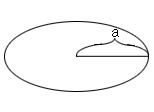A# semimajor axis

The semimajor axis is half the longest distance (major axis) across an ellipse. The semimajor axis is one of the orbital elements – a standard parameter used to describe an elliptical orbit. The semimajor axis is also the average distance of an orbiting object from its primary. The periapsis and apoapsis distances, rp and ra, can be calculated from the semimajor axis, a, and the eccentricity, e, by the formula

rp = a(1 - e) and ra = a(1 + e)semimajor axis